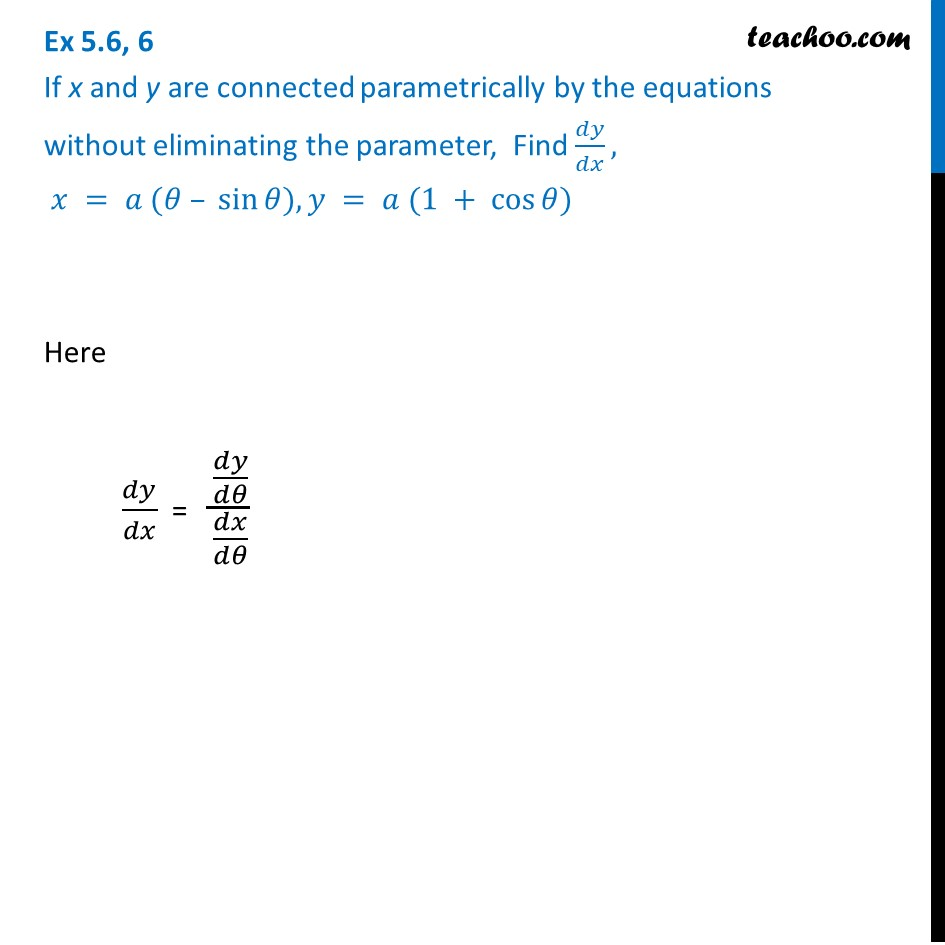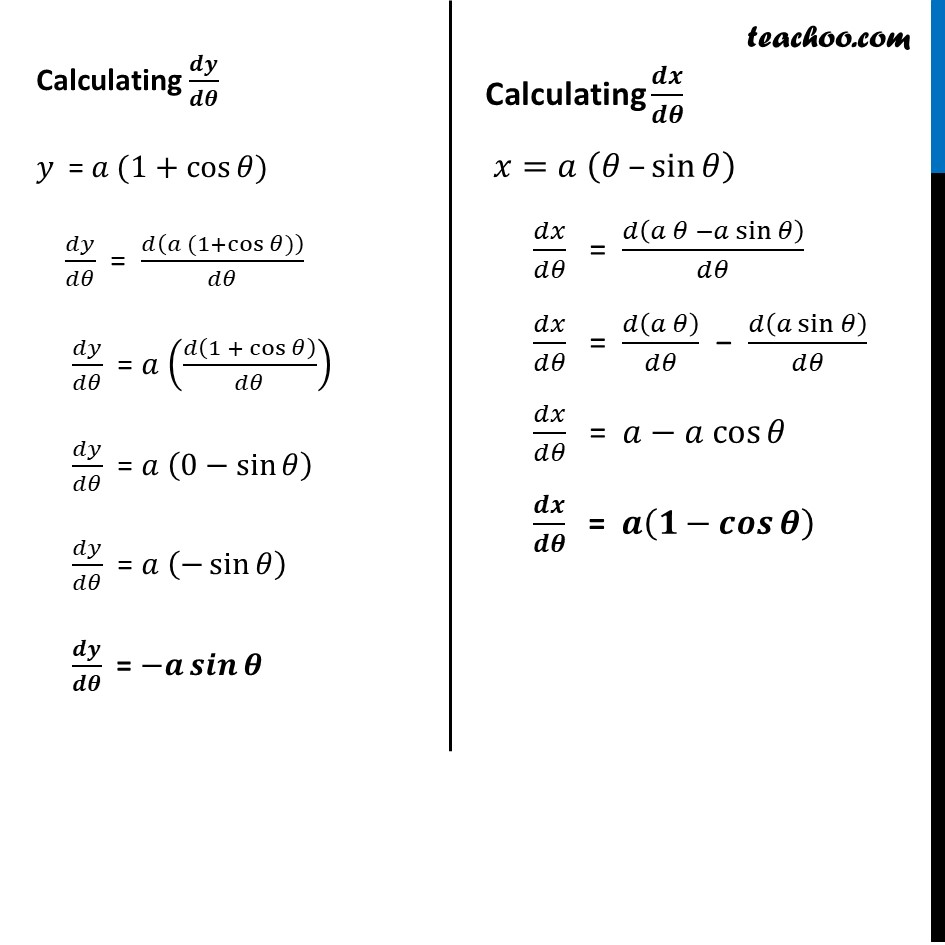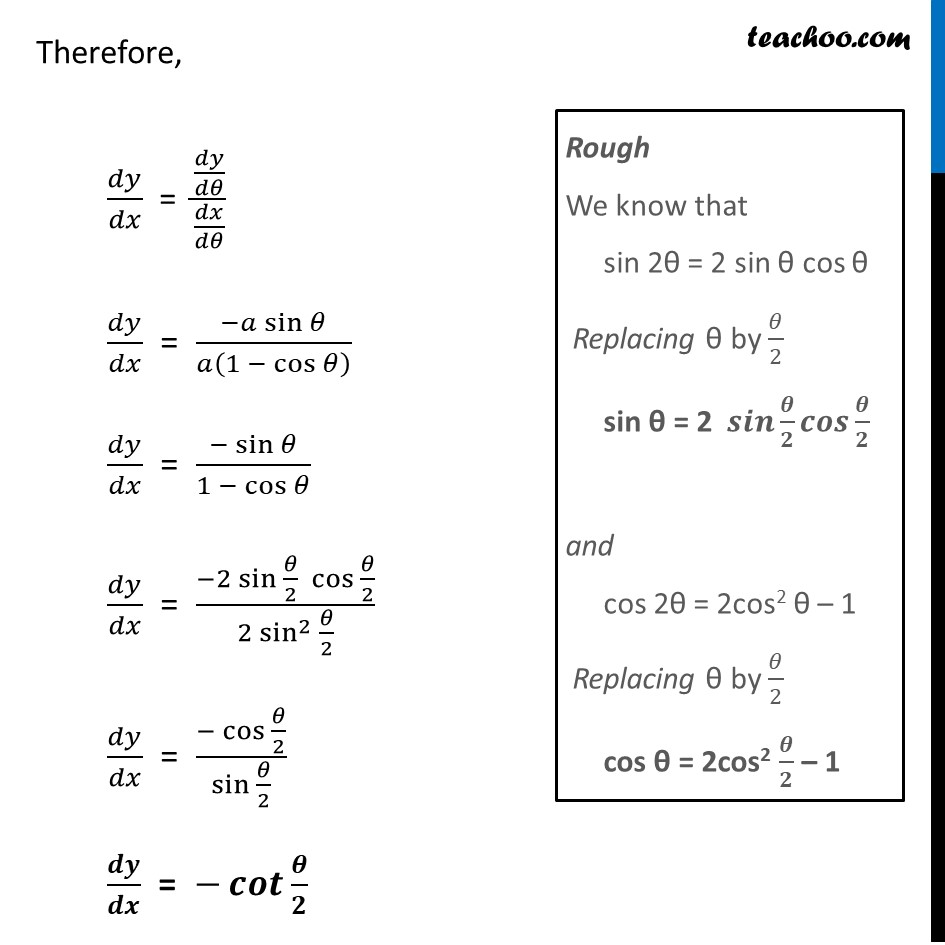Derivatives in parametric form

Chapter 5 Class 12 Continuity and Differentiability
Concept wiseLearn in your speed, with individual attention - Teachoo Maths 1-on-1 Class

### Transcript

Ex 5.6, 6 If x and y are connected parametrically by the equations without eliminating the parameter, Find 𝑑𝑦/𝑑𝑥, 𝑥 = 𝑎 (𝜃 – sin⁡𝜃), 𝑦 = 𝑎 (1 + cos⁡𝜃)Here 𝑑𝑦/𝑑𝑥 = (𝑑𝑦/𝑑𝜃)/(𝑑𝑥/𝑑𝜃) Calculating 𝒅𝒚/𝒅𝜽 𝑦 = 𝑎 (1+cos⁡𝜃) 𝑑𝑦/𝑑𝜃 = 𝑑(𝑎 (1+cos⁡𝜃))/𝑑𝜃 𝑑𝑦/𝑑𝜃 = 𝑎 (𝑑(1 + cos⁡𝜃 )/𝑑𝜃) 𝑑𝑦/𝑑𝜃 = 𝑎 (0−sin⁡𝜃 ) 𝑑𝑦/𝑑𝜃 = 𝑎 (−sin⁡𝜃 ) 𝒅𝒚/𝒅𝜽 = −𝒂 𝒔𝒊𝒏⁡𝜽 Calculating 𝒅𝒙/𝒅𝜽 𝑥=𝑎 (𝜃 –sin⁡𝜃 ) 𝑑𝑥/𝑑𝜃 = 𝑑(𝑎 𝜃 −𝑎 sin⁡𝜃 )/𝑑𝜃 𝑑𝑥/𝑑𝜃 = 𝑑(𝑎 𝜃)/𝑑𝜃 − 𝑑(𝑎 sin⁡𝜃 )/𝑑𝜃 𝑑𝑥/𝑑𝜃 = 𝑎−〖𝑎 cos〗⁡𝜃 𝒅𝒙/𝒅𝜽 = 𝒂(〖𝟏−𝒄𝒐𝒔〗⁡𝜽 ) Therefore, 𝑑𝑦/𝑑𝑥 = (𝑑𝑦/𝑑𝜃)/(𝑑𝑥/𝑑𝜃) 𝑑𝑦/𝑑𝑥 = (−𝑎 sin⁡𝜃)/𝑎(〖1 − cos〗⁡𝜃 ) 𝑑𝑦/𝑑𝑥 = (−sin⁡𝜃)/〖1 − cos〗⁡𝜃 𝑑𝑦/𝑑𝑥 = (−2 〖sin 〗⁡〖𝜃/2〗 〖cos 〗⁡〖𝜃/2〗)/(2 〖sin^2 〗⁡〖𝜃/2〗 ) 𝑑𝑦/𝑑𝑥 = (−〖cos 〗⁡〖𝜃/2〗)/(sin 𝜃/2) 𝒅𝒚/𝒅𝒙 = −𝒄𝒐𝒕⁡〖𝜽/𝟐〗 Rough We know that sin 2θ = 2 sin θ cos θ Replacing θ by 𝜃/2 sin θ = 2 𝒔𝒊𝒏⁡〖𝜽/𝟐〗 𝒄𝒐𝒔⁡〖𝜽/𝟐〗 and cos 2θ = 2cos2 θ – 1 Replacing θ by 𝜃/2 cos θ = 2cos2 𝜽/𝟐 – 1